﻿ History of Atomic Theory

#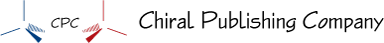History of Atomic Theory

When I was writing my text, I decided that I wanted to devote more time and space to describing the modern model of the atom instead of including the traditional description of the historical development of atomic theory. The purpose of this webpage is supplement what I did in the text by describing this history.

Our story starts with two of the scientific laws suggested in the late 18th and early 19th centuries. Scientific Laws are general statements about nature that are based on repeated experiments or observations. One way to distinguish between scientific laws and scientific theories is that scientific laws describe what happens, and scientific theories explain why things happen.

The first scientific law that affected the development of atomic theory is the Law of Conservation of Mass (or Matter) that states that matter and thus mass are neither created nor destroyed in the process of normal chemical reactions. (The modern version qualifies this a bit.) The second scientific law that concerns us is the Law of Definite Proportions (or Constant Composition) that states that a specific compound always contains the same elements in the same definite proportions by mass. The importance of these laws for us is that they provided 19th and 20th century scientists with the challenge of how to explain them.

With help from other scientists, in the early 19th century, John Dalton developed a theory that can be used to explain the Law of Conservation of Mass and the Law of Definite Proportions. Dalton's Atomic Theory states that

1. Elements are composed of tiny, indivisible particles of matter called atoms.

2. Atoms of the same element have the same mass, and atoms of different elements have different masses. (It was later found that isotopes of the same element can have atoms with different masses.)

3. Chemical reactions involve the separation and combination of atoms, but the atoms themselves are never created, destroyed, or even changed.

4. Compounds are composed of combinations of atoms of two or more elements with the atoms combining in a specific ratio with respect to the number of atoms of each element.

Dalton’s Atomic Theory can be used to explain the Law of Conservation of Mass. In Dalton's view, chemical reactions involve a rearrangement of the atoms, but the atoms themselves are conserved. Because the atoms carry the mass, and because the atoms are conserved, the mass is conserved.

Dalton’s Atomic Theory can also be used to explain the Law of Definite Proportions. Because compounds are always composed of the same elements in specific ratios with respect to the number of atoms of each element, and because atoms of the same element have the same mass and atoms of different elements have different masses, specific compounds must be composed of the same elements in the same definite proportion by mass.

Dalton was not able to provide an adequate explanation for why atoms of one element are different from another. It was improbable that size or mass alone could account for the widely varying chemical and physical properties of the elements. For example, his theory could not explain why lithium and sodium are so similar when sodium is almost three times heavier than lithium or why elements that are closer size, such as lithium and helium are so different.

The clue to the explanation of the differences among atoms of different elements lay in the discovery of the internal structure of the atom. A series of experiments done in the late 19th century and the early 20th century showed that atoms are composed of protons, neutrons, and electrons.

J. J. Thomson is generally given credit for the discovery of the electron, but like with most scientific discoveries, the work of others made his discovery possible. Science is a team sport. I’ll just describe a few of the steps that led to Thomson’s work. As we will see, Thomson based his theory of the atom in part on experiments done with a cathode ray tube, which was developed by the glassblower Heinrich Geissler and German physicist Julius Plücker in the 1850s. A cathode ray tube consists of a sealed tube that has most of the air pumped out of it. There are two electrodes in the tube, and a strong voltage sends an electric current between the electrodes. Plücker observed a green glow on the wall of the tube and suggested that it was due to a ray coming from the cathode. In 1879, William Crookes found that the cathode rays were deflected by a magnetic field, so he postulated that the rays were composed of negatively charged particles. Crookes also showed that the results of his experiments were independent of the nature of the gas in the tube.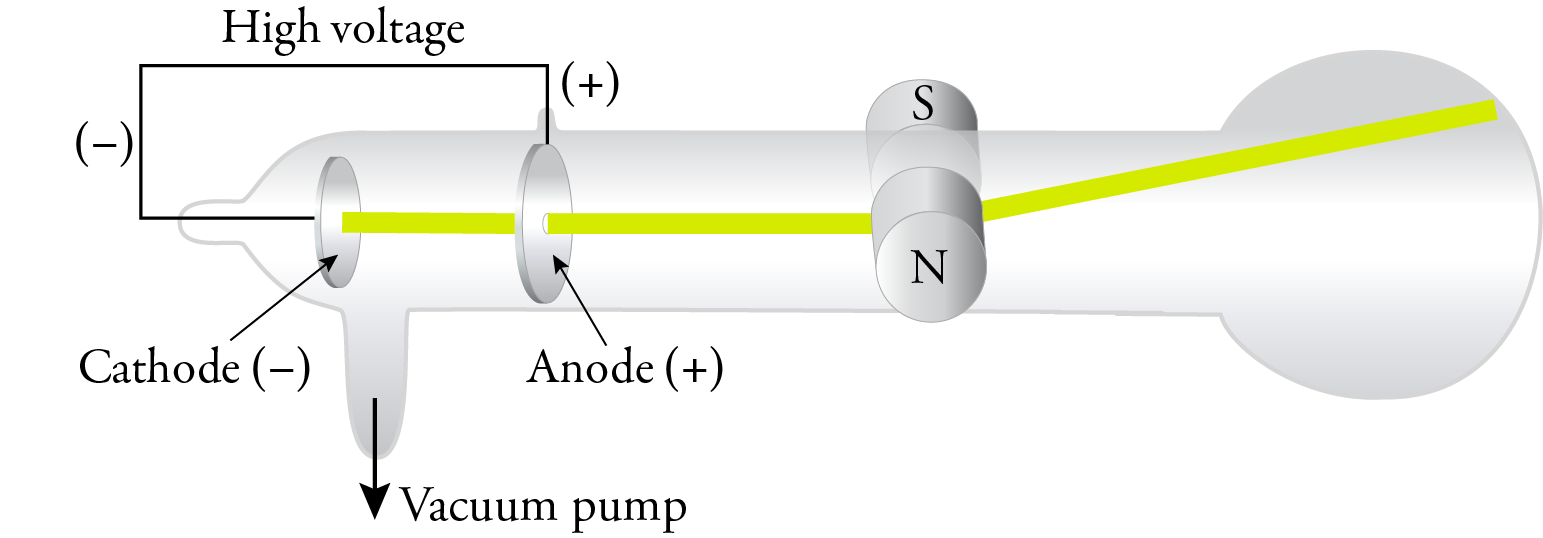In 1897, J. J. Thomson replaced the magnetic field with an electric field in a cathode ray tube with an improved vacuum, and he showed that the cathode rays were attracted to the positive plate and repelled by the negative plate. This confirmed that the cathode rays were composed of negatively charged particles. Thomson called these particles corpuscles, but they have come to be called electrons, a name suggested by George Johnstone Stoney in 1891.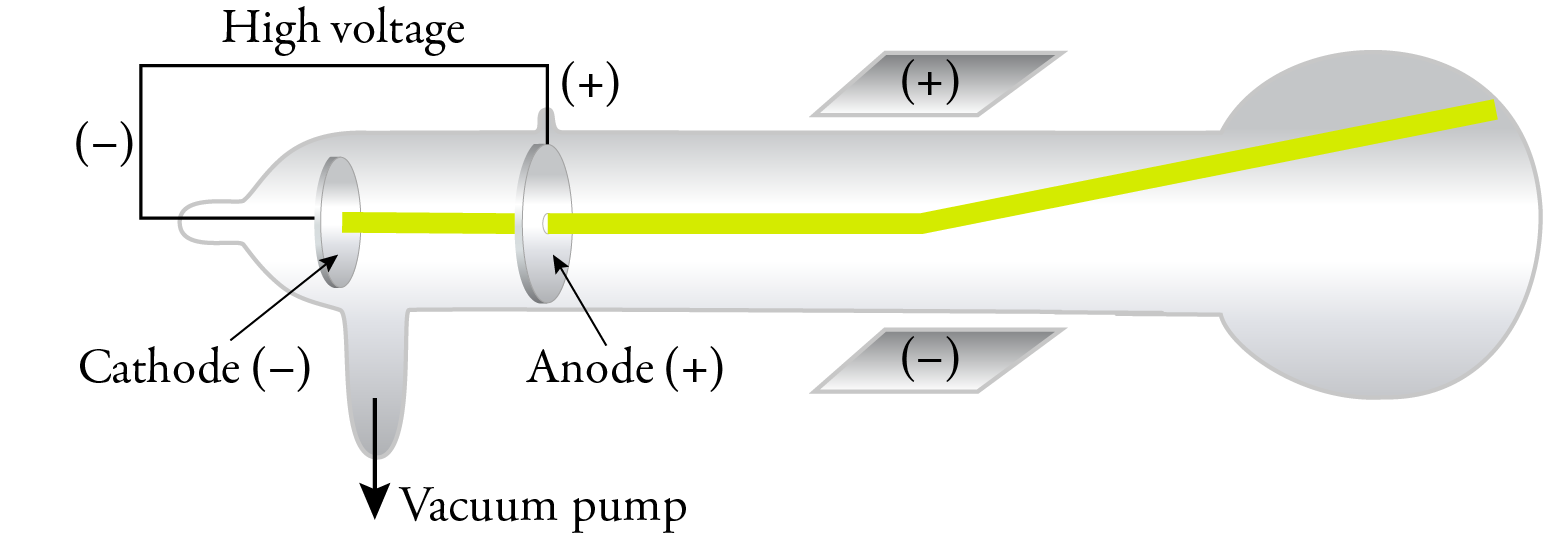Thomson was able to calculate the mass to charge ratio, and the results of his experiments and calculations suggested that electrons were about 1/1000 the mass of hydrogen atoms, suggesting that atoms are composed of particles smaller than the atoms itself. Because the results of his experiments were independent of the gas in the tube, he concluded that all substances contain these same electrons.

In 1909, Robert Millikan and Harvey Fletcher observed charged oil droplets falling between two electrically charged plates. They were able to determine the overall charge on the droplets, and they calculated that all of these charges were integral multiples of 1.59×10−19 coulomb (now calculated to be 1.602176634×10−19 coulomb). Millikan assumed that this was the charge of an electron, and from the mass to charge ratio determined by Thomson, the mass of the electron was found to be about 1/1836 the mass of a hydrogen atom, confirming that atoms are composed of particles much smaller than even the smallest atom.

Thomson suggested the first model of the atom to consider this internal structure. Because atoms are uncharged overall, he knew that there must be positively charged material in each atom to balance the electrons’ negative charge, and because the positive material must be much more massive than the corpuscles (electrons), he suggested that the positive material occupies most of the volume of the atom. He postulated that the tiny electrons were widely spaced in the atom, like raisins in a plum pudding, so we call his model the Plum Pudding model.There are three important players in in the next step for atomic theory. Ernst Rutherford was a professor of physics and the Victoria University of Manchester (now the University of Manchester). He was one of the pioneers in the study of radioactivity. Rutherford invited the German physicist Hans Geiger to help him with his research, and Ernest Marsden was an undergraduate physicist studying under Geiger.

At the suggestion of Rutherford, Geiger and Marsden did a series of experiments that we call the gold foil experiments. They directed a beam of alpha particles at a thin piece of gold foil and determined the path of these alpha particles after they struck the foil. Alpha particles are tiny, positively charged particles emitted by some radioactive substances. In the 1909 version of Geiger and Marsden’s experiment, they used radioactive radon, radium, and bismuth-214 to generate the alpha particles.

If Thomson’s plum pudding was correct, because of the balance of positive and negative charges and diffuse arrangement of these charges, the alpha particles should have passed through the foil almost unaffected.This is not what they found. Although most of the particles did go through the foil unaffected, some of them were deflected more than expected, and, most surprisingly, a very small fraction of the alpha particles was deflected back toward the source.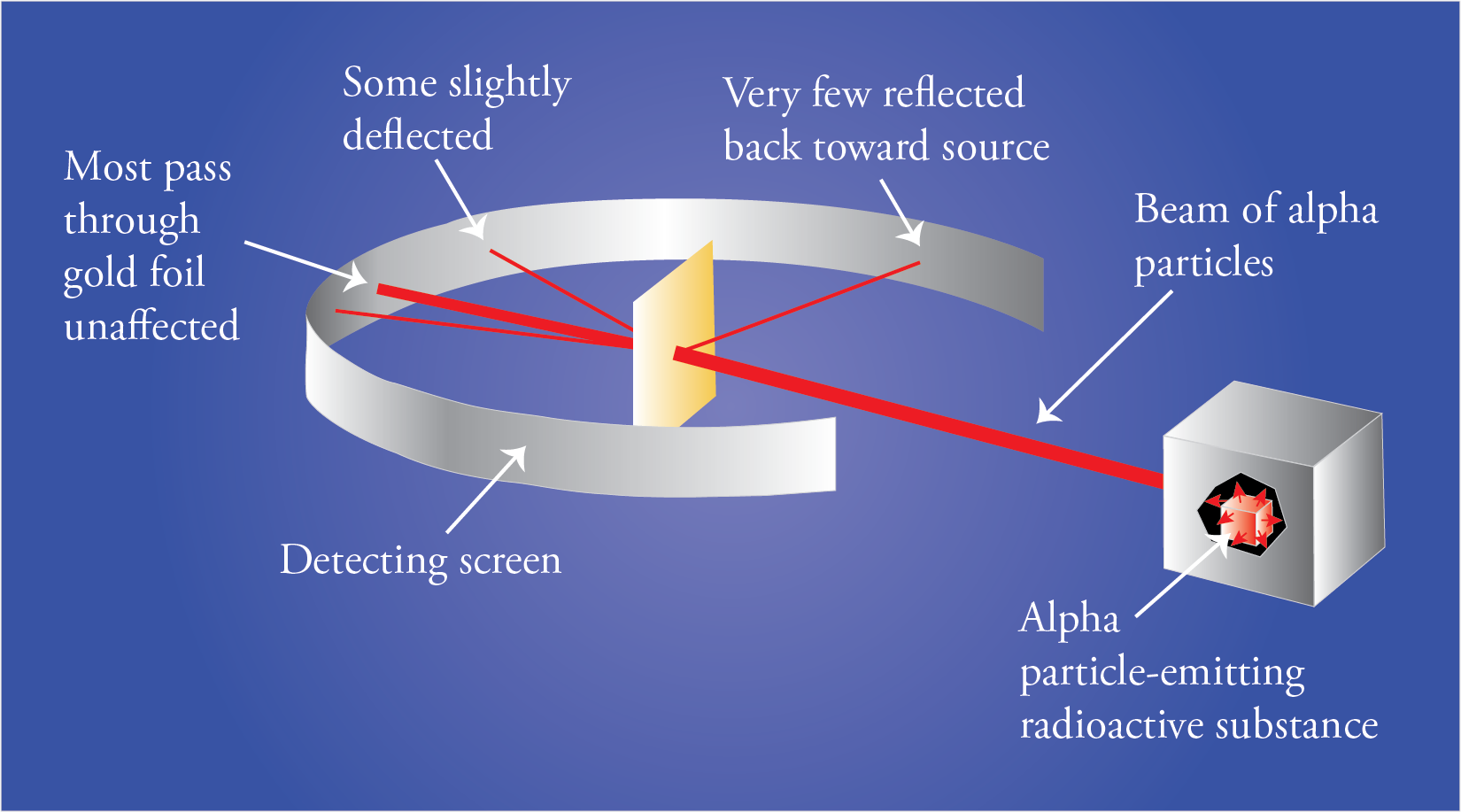The results of the experiment led Rutherford to postulate a new model for the atom. To explain the results of the gold foil experiment, he suggested that the atom must be mostly empty space with most of the mass and all of the positively charged particles (protons) in a tremendously small and tremendously dense core of the atom that we now call the nucleus. We now know that the diameter of the nucleus is about 1/100,000 the diameter of the atom. This very high density of positive charge provides the repulsive force that would be necessary to stop an alpha particle and accelerate it back toward the source. According to Rutherford, most of the particles went through unaffected because most of the volume of the atom is empty. These particles never encountered any proton or electron material. The particles that came close to a nucleus but did not have head-on collisions were deflected a little bit, and the particles that had head-on collisions with nuclei were stopped by the plus-plus repulsion and accelerated back toward the source.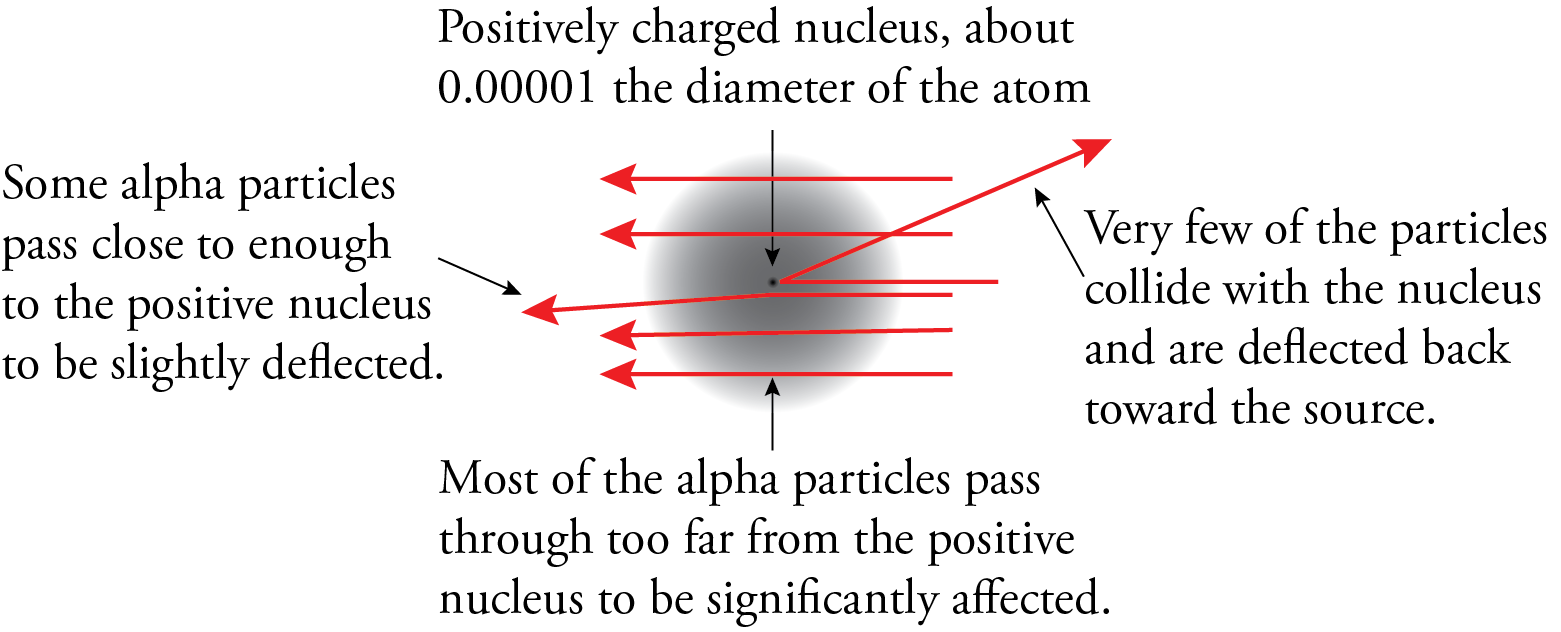Rutherford did not focus much of his attention on electrons, but he did recognize that because the nucleus had to be positive to explain the behavior of the alpha particles in the gold foil experiment, the electrons could not be in the nucleus. Rutherford thought that the electrons had to be moving to keep them from being attracted into the nucleus, so he suggested that the electrons were orbiting around the nucleus like planets around the sun. For this reason, his model is often called the planetary model of the atom. (Don’t get attached to this view of electrons as orbiting the nucleus because we don’t think that’s the way electrons act.)

There were some problems with the planetary model. An orbiting charged particle, such as the electron, should constantly lose energy, decrease the radius of its orbit, and collapse into the nucleus. Also, the model doesn't explain the similarities and differences between the elements. Why are sodium and lithium so similar? Why are fluorine and neon so different?

Niels Bohr provides us with the next model of the atom, but before I describe his model, let’s look at some things that affected his conclusions. We start with a description of the nature of radiant energy. Radiant energy (or light) appears to have a dual nature, particle and wave. It’s wave character can be described in terms of an oscillating electric field perpendicular to an oscillating magnetic field. Because of this, it is often called electromagnetic radiation.The waves of different forms of radiant energy vary with respect to wavelength and frequency. Wavelength, which is described with the symbol λ, is the distance between two like points on the wave or the length of the period of the wave. A period is the portion of the wave for which it has gone through all the changes in magnitude and direction of its electric (or magnetic) field and returned to where it started. Frequency is the number of periods that pass a point per second.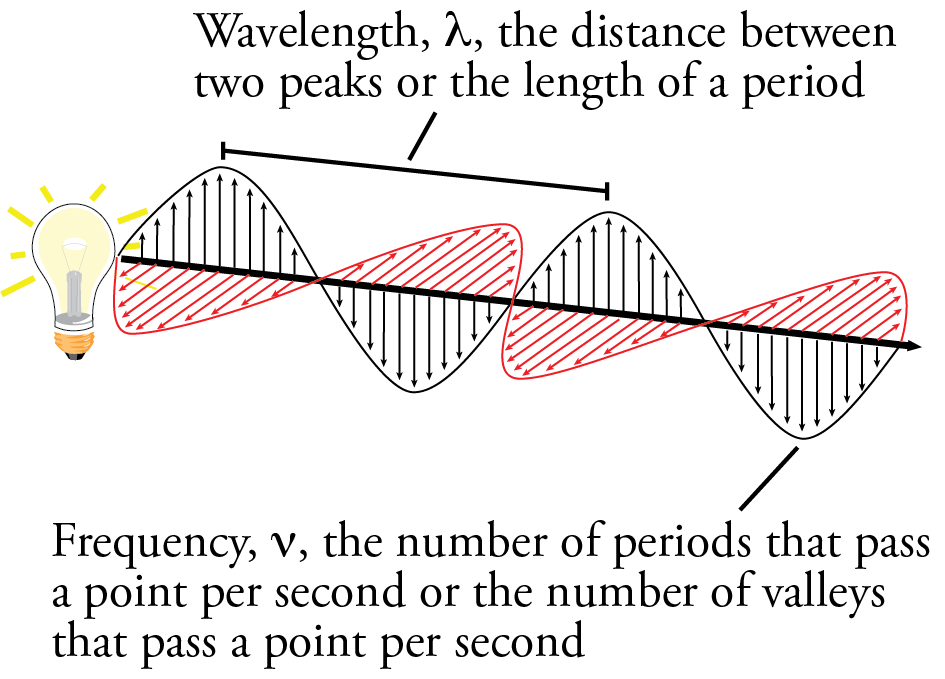Wavelength and energy are inversely proportional. The longer the wavelength is, the lower the energy. The shorter the wavelength is, the higher the energy. Frequency and energy are directly proportional. The higher the frequency is, the higher the energy. The lower the frequency is, the lower the energy.

Radiant energy is most often a mixture of rays of different wavelengths. We can separate rays of different wavelengths with a spectroscope. The spectroscope has three main parts. (1) It has a slit that allows a narrow beam of light to enter. (2) It has a prism or diffraction grating that separates the light by wavelength. The shorter the wavelength, the more it is refracted from its straight-line path. Refraction is the bending of waves as they pass from one medium into another with a different density. (3) It has a lens to allows the light to be focused.

When a metal is heated to high temperature, it gives off a continuous light spectrum. A continuous spectrum includes all wavelengths of radiant energy. The continuous visible light spectrum is shown below.We classify the different parts of the continuous spectrum using two criteria: (1) the source of the radiant energy and (2) the effect on the physical world. The following table shows the divisions of the continuous light spectrum.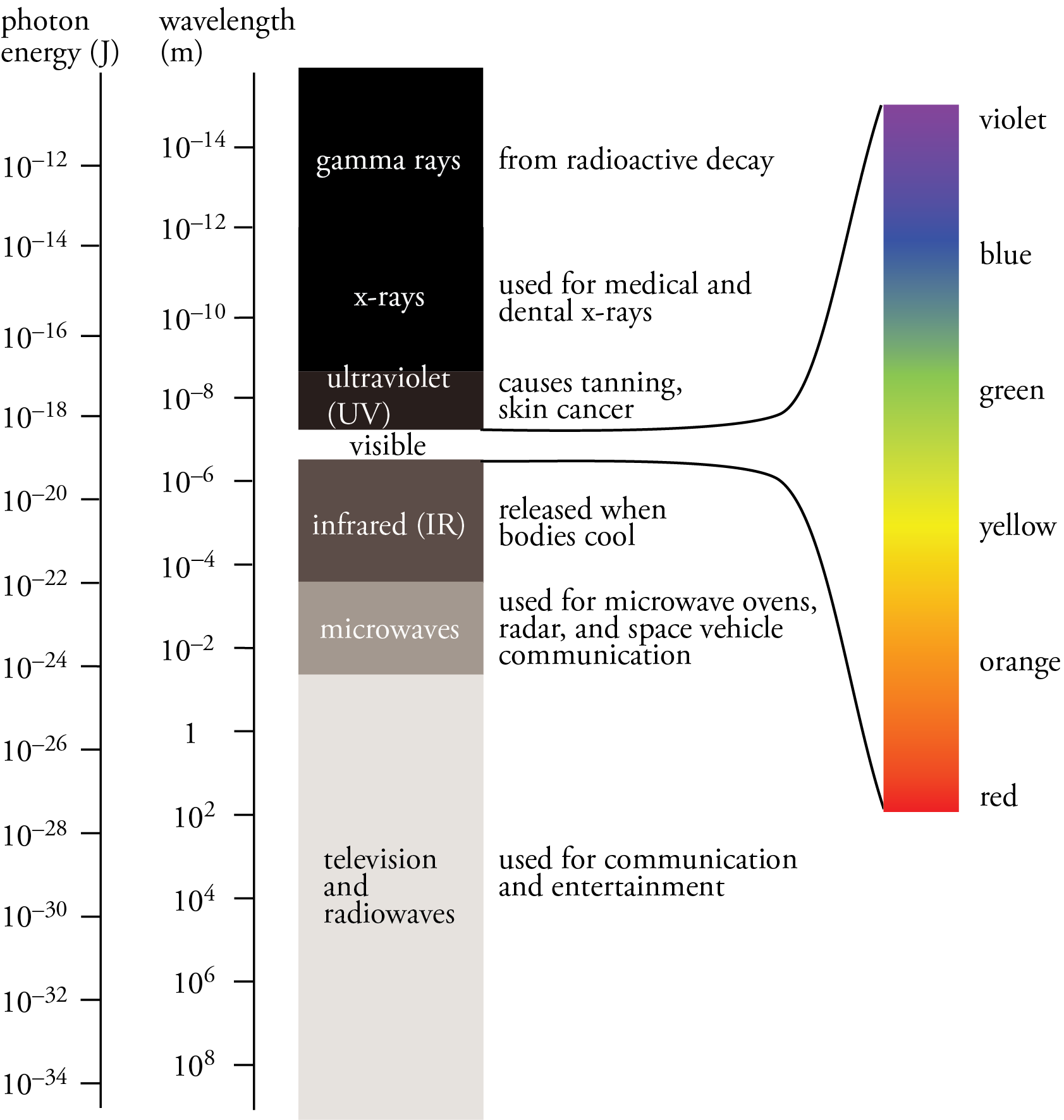When a gaseous element is heated to high temperature, and the light is passed through a spectroscope, a line spectrum is observed. The following is the line spectrum in the visible light range for hydrogen. The lines are red, blue-green, and violet.J. J. Balmer observed the visible portion of the line spectrum of hydrogen in 1885, and he noticed a pattern. He found he was able to calculate the wavelengths of the lines in the line spectrum above from an equation he developed. The following equation is a modern equivalent to Balmer’s equation, and it is in a form that will help me to make a point soon.Balmer was not able to explain why the equation worked. He could only show that it fit experimental observations. Because of Balmer’s work, we now call the collection of lines for hydrogen that lie in the visible range the Balmer Series.

Theodore Lyman saw a similar pattern in the ultraviolet portion of the hydrogen line spectrum. He developed an equation that was similar to the Balmer equation that allowed him to calculate the wavelength in the UV range. Again, the following equation is a modern equivalent to Lyman’s equation, written in a form that will help me to make a point soon. It contains the same Rydberg constant as the Balmer equation. The lines in the ultraviolet region of the hydrogen line spectrum are now called the Lyman Series.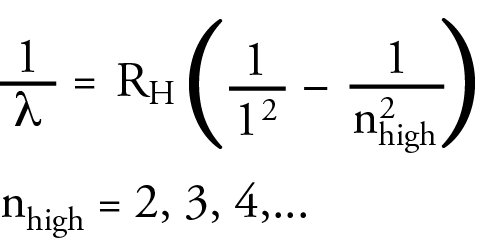Friedrich Paschen saw a similar pattern in the infrared portion of the hydrogen line spectrum. The wavelength in the IR region could be calculated with an equation that was similar to the Balmer equation. Again, the following equation is a modern equivalent to Paschen’s equation. The lines in the infrared region of the hydrogen line spectrum are now called the Paschen Series.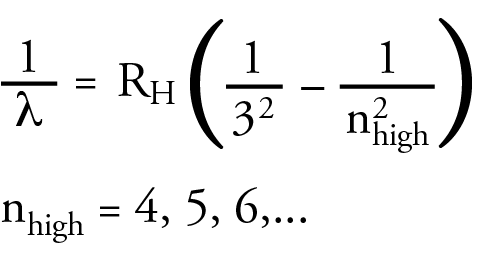Rydberg summarized the three equations in the general equation below.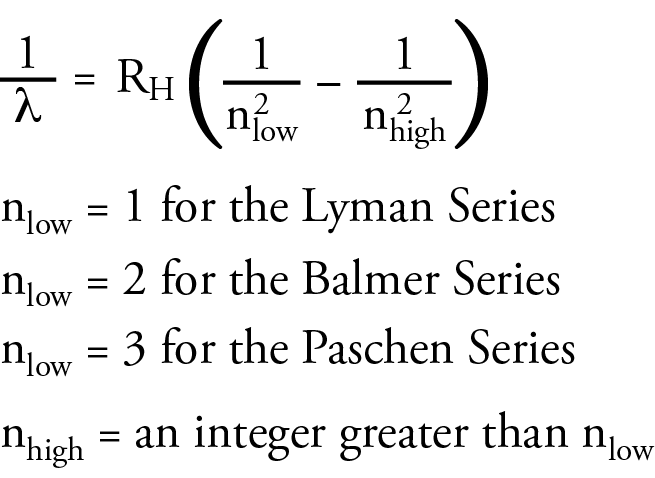None of these scientists could explain why these equations worked. Their main contribution to the development of atomic theory was to propose a challenge to other scientists to explain this.

As I mentioned, light has a dual character, particle and wave. The combined works of Max Planck, Albert Einstein, and others led to the conclusion that radiant energy is composed of small packets of energy called photons.

Niels Bohr suggested a model for the atoms to explain the following questions.

1. Why do we get a line spectrum when we heat a gaseous element?

2. What is the significance of nlow and nhigh in the Rydberg equation?

3. Why is the Lyman Series (UV) higher energy than the Balmer Series (visible), and why is the Balmer Series higher energy than the Paschen Series (IR)?

4. Why aren't electrons losing energy steadily and spiraling into the nucleus?

5. What makes one element similar to or different from another?

6. How are photons created?

Here are the fundamental components of the Bohr model of the atom.

1. The atom has most of its mass and all the positive charge in a tremendously dense core. (He accepted Rutherford’s nuclear model.)

2. Electrons revolve around the nucleus in specific stable orbits with specific radii. They can maintain these stationary orbits without radiating energy, and they cannot have any orbit between these stationary orbits.

3. There is a specific potential energy associated with each allowed stationary orbit. This energy can be called an energy level. Because only certain radii of orbits are allowed, only certain energies are allowed. The energy levels are associated with integer numbers starting with 1, e.g. n=1,2,3,…

4. The radii of the orbits and therefore the energies for the energy levels get closer and closer together as the numbers for the energy levels increase.

5. Electrons can only gain or lose energy by moving from one allowed orbit or energy level to another. When an electron moves from a higher energy level to a lower energy level, it releases a photon with an energy equal to the energy difference between these energy levels.

6. Each orbit or energy level can only have a maximum of 2n2 electrons. Therefore, the n=1 energy level can contain a maximum of 2 electrons, the n=2 energy level can contain a maximum of 8 electrons, the n=3 energy level can contain a maximum of 18 electrons, the n=4 energy level can contain a maximum of 32 electrons, the n=5 energy level can contain a maximum of 50 electrons, the n=6 energy level can contain a maximum of 72 electrons, etc.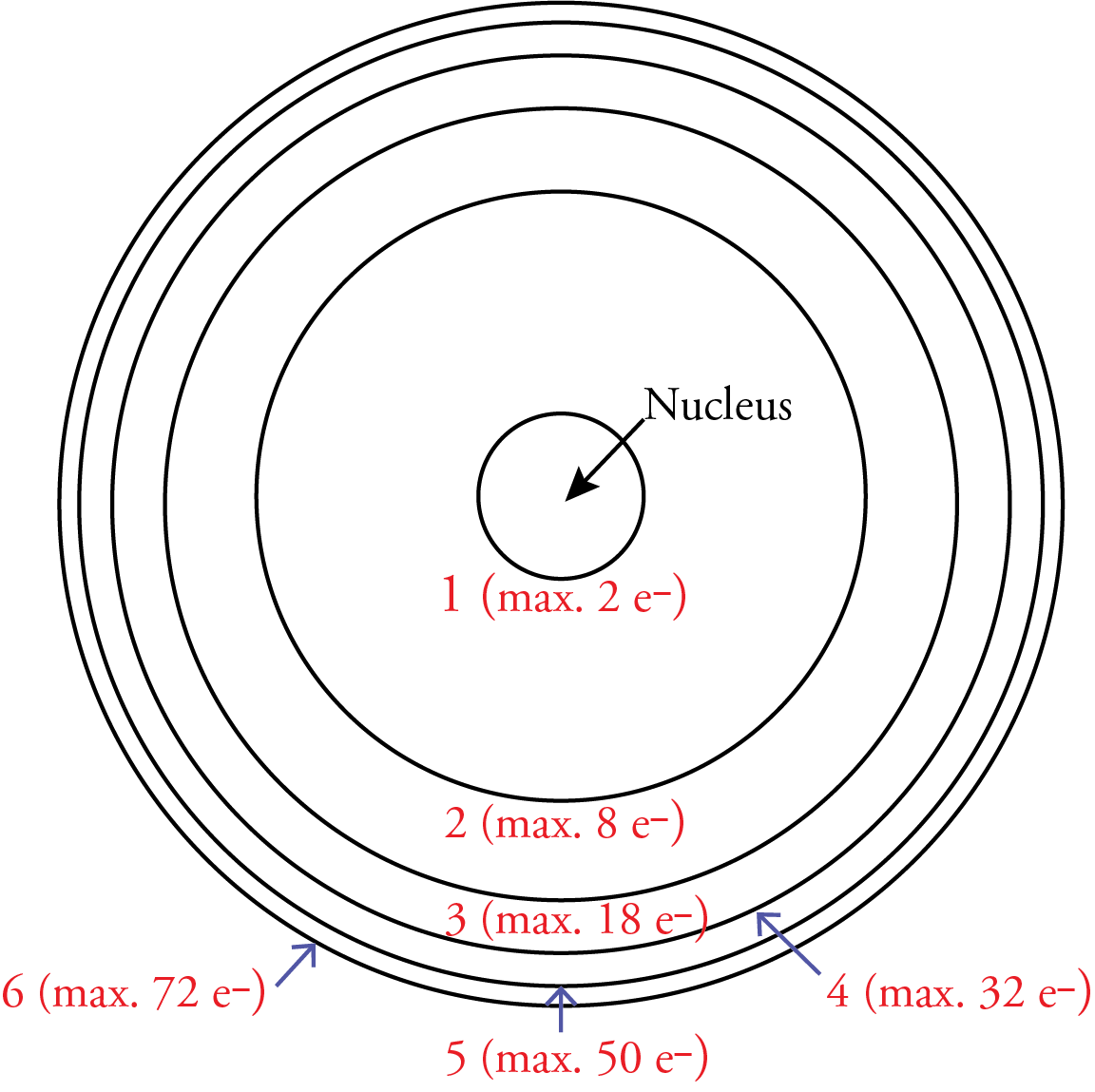Whenever a scientist suggests a new scientific model, there are always controversies, and Bohr’s model of the atom is no exception, but there are several reasons why the scientific community took Bohr’s model seriously. First, it was the first model that explained why the Rydberg equation could be used to calculate the wavelengths of the lines in the hydrogen line spectrum.According to the Bohr model, nlow was the energy level an electron is moving to when it moves from a higher energy level to a lower energy level. The nhigh in the Rydberg equation represents the energy level the electron is moving from. For the Lyman series, ultraviolet photons are released when an electron moves from a higher energy level to the n=1 energy level. For the Balmer series, visible photons are released when an electron moves from a higher energy level to the n=2 energy level. For example, when an electron moves from the n=3 energy level to the n=2 energy level, it releases a photon with the energy difference between these energy levels, which our eyes and brains associate with the color red. When an electron moves from the n=4 energy level to the n=2 energy level, it releases a higher energy photon with the energy difference between these energy levels, which our eyes and brains associate with the color blue-green. Because the energy levels are getting closer together with increasing n, when an electron moves from any energy level higher than 4 down to the n=2 energy level, it releases a photon that our eyes and brains associate with the color violet. For the Paschen series, infrared photons are released when an electron moves from a higher energy level to the n=3 energy level.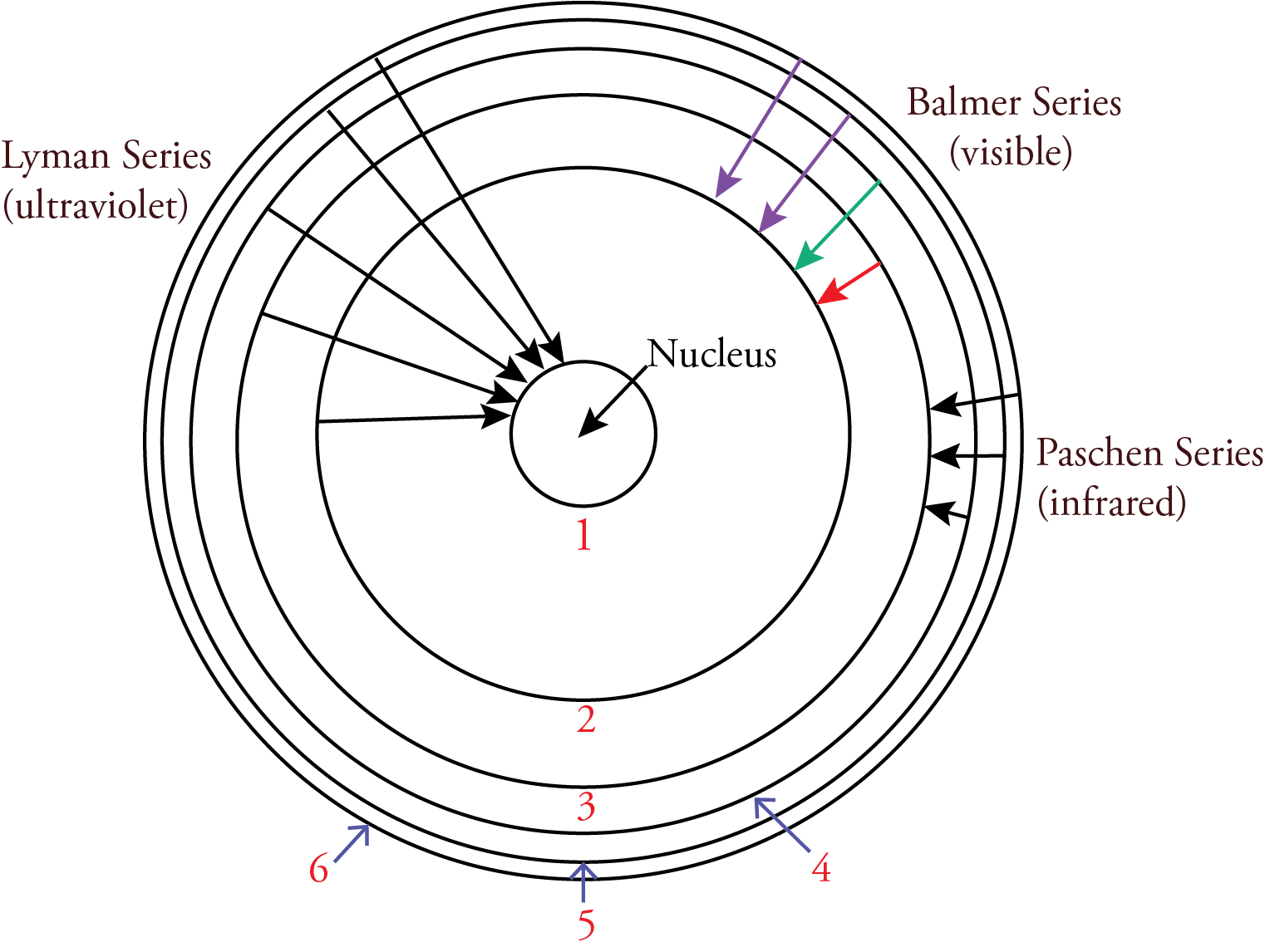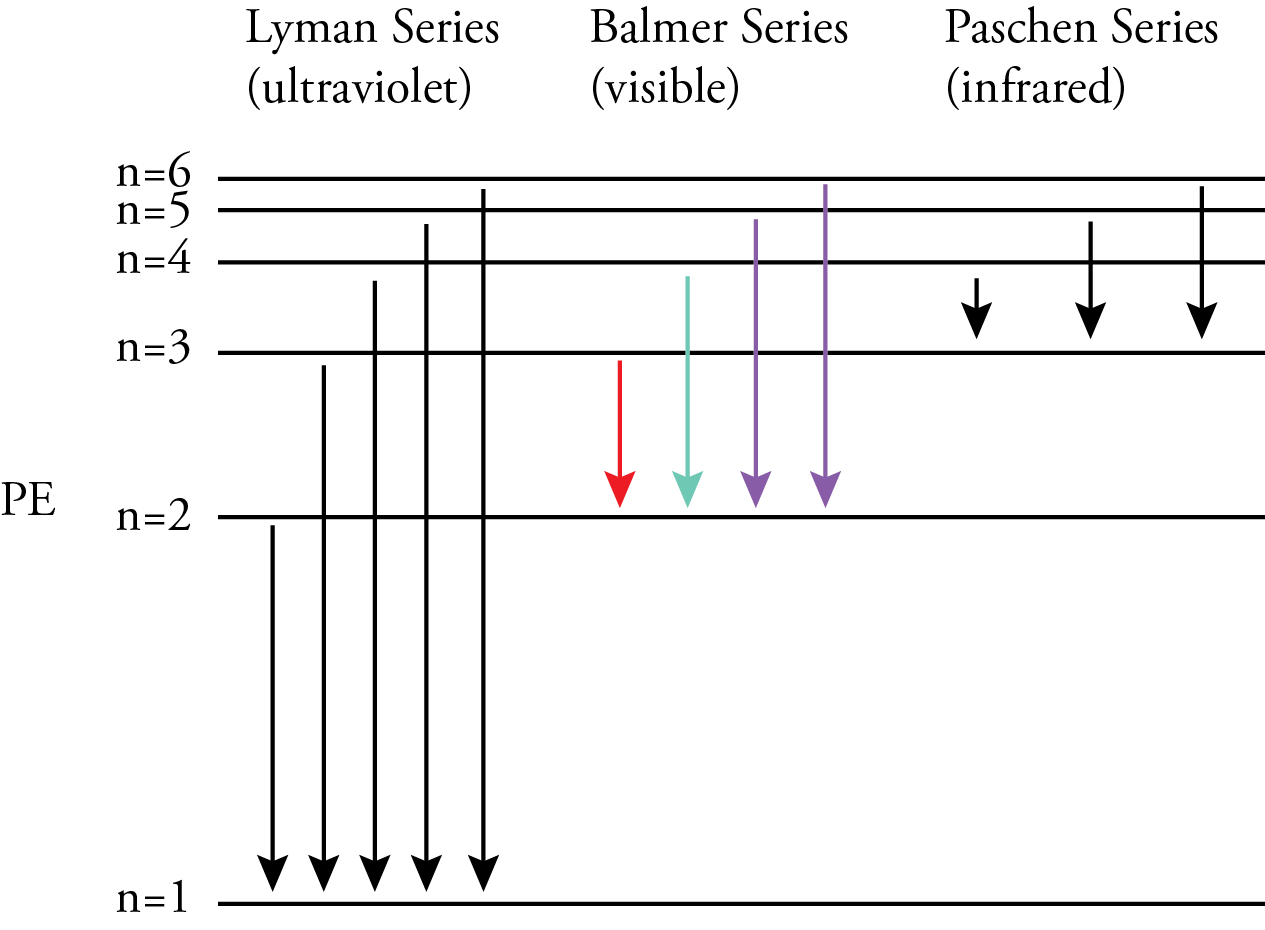Bohr recognized that if his model was correct, an electron must move from one energy level to another without ever having the energies in-between. This was a big problem since that would mean that an electron would have to move from one circular orbit to another without ever being in-between. The conventional view of particles does not allow this, so Bohr concluded that we must renounce all attempts to visualize or describe the behavior of the electrons as they move from one stationary state to another.

The following describes Bohr's answers to some of the questions of his day.

What is the source of light? Photons of light are released when an electron moves from a higher energy level to a lower energy level. The energy of the photon is equal to the energy difference between the energy levels.

Why do we get a line spectrum when we heat a gaseous element? The energies allowed an electron in an atom are limited to certain specific values or energy levels. When electrons are heated to high temperature, they can be excited to higher energy levels. In a sample containing many atoms, electrons can be excited to all possible energy levels. The excited electrons fall back down and release light photons with energy equal to the difference in energy between the energy levels. In a sample containing many atoms, all of the possible jumps in energy will be represented. Specific energy levels lead to specific differences in energy and lead to specific energies of photons. The specific energies of the photons are associated with specific frequencies and wavelengths. If the radiant energy is in the visible portion of the light spectrum, it will be of specific color.

The Bohr model could explain the similarities and differences between the elements through chlorine. (His model cannot be used to explain the similarities and differences between the elements beyond chlorine.) Bohr assumed that the lowest energy levels would be filled first, and when an energy level is filled, the next electrons would begin to fill the next higher energy level. For example, the two elecrons for helium would have the Bohr configuration of 2e) (with two electrons filling the first energy level), and the 10 electrons of neon would have the Bohr configuration of 2e)8e) (with two electrons filling the first energy level and eight filling the second energy level). This shows why helium and neon are chemically similar. Their highest occupied energy level is filled. As another example, the three electrons of lithium would have the Bohr configuration of 2e)1e (with two electrons filling the first energy level and one electron in the second energy level), and the 11 electrons of sodium would have the Bohr configuration of 2e)8e)1e (with two electrons filling the first energy level, eight filling the second energy level, and one electron in the third energy level). Lithium and sodium are chemically similar because they both have one electron in their highest occupied energy level. Similar reasoning could be used to explain why fluorine, 2e)7e, and chlorine, 2e)8e)7e, are so similar. We need the modern model of the atom can explain the similarities and differences of the elements past chlorine.

Louis De Broglie provided the next step in our understanding of matter. His ideas helped to answer some of the questions left by the Bohr model. In 1924, De Broglie postulated that (1) matter has wave character, (2) that the smaller the particle is, the greater the wave character, and (3) that the electron has significant wave character. De Broglie was able to calculate the wavelength of a moving particle. The De Broglie wavelength of an electron moving at 10% the speed of light is 0.024 nm, showing that the tiny electron has a significant wave character. (The De Broglie wavelength of 7.41x10-26 nm for a 200 g baseball moving at 100 miles per hour or 44.7 m/s shows that the wave character of larger particles is insignificant.)

There is experimental evidence that the electron has wave character. If light waves are directed at a wall with two slits in it, a diffraction pattern is found on the far side of the wall. A diffraction pattern is a series of light and dark sections to the right and left of the slits, and it can only be explained in terms of waves. When a beam of electrons is directed at a crystal structure, a diffraction pattern is found on the far side of the crystal. This series of areas where electrons are found or not found can only be explained by accepting that electrons have wave character.

After De Broglie's surprising concept of the electron as a wave, there were many questions. What is the electron really? Is it a wave, a particle, or some blend of the two? Where is the electron in the atom? How is it moving? Werner Heisenberg tried to think of how we might answer these questions. An accurate description of Heisenberg’s conclusions requires delving into quantum mechanics more than we want to, so here’s a simplified version of the issues he recognized that I think will give you a glimpse at his conclusions.

One way to find an electron would be to send a photon into the atom and bounce it off the electron. We could determine where the electron was from the direction from which the photon returns. If we were to do this many times, the seems that we could reconstruct the motion of the electron. One problem with this is that like all measurements, there would be some uncertainty in determining where the returning photon is returning from. To minimize this uncertainty, it would be best to use a photon associated with a very short wavelength, but there is a problem with this too. When a photon strikes an electron, it can move it. The shorter the wavelength, the higher the energy of the photon and the more it will move the electron, making it more uncertain that the position where you find it the second time is the position it would have been if you had left it alone the first time. This leads to uncertainty in how the electron is moving. Thus, if you want to know how the electron is moving, it seems best to use a low energy, long wavelength photon that would not move the electron much, but this would lead to greater uncertainty in determining the position of the electron. This conflict between wanting to minimize the uncertainty in the position with a short wavelength, high energy photon and wanting to minimize the uncertainty in the motion by using a low energy, long wavelength photon leads to the conclusion that we would never know exactly where the electron is and how it is moving at the same time.

In 1927, Werner Heisenberg concluded that the more precisely the position of an electron is determined, the less precisely its momentum (mass times velocity) can be determined, or the more precisely the momentum is determined, the less precisely the position of the electron can be determined. This statement is called the Uncertainty Principle or commonly the Heisenberg Uncertainty Principle. It is thought to be a fundamental characteristic of matter waves.

The Uncertainty Principle led many scientists to relax their quest to describe the true nature of the electron. Erwin Schrodinger and others accepted De Broglie's assumption that the electron has wave character and applied the known three-dimensional wave mathematics to the electron wave of a hydrogen atom, which led to the modern model of the atom. See Chapter 4 of the atoms-first version of my text or Chapter 11 of the chemistry-first version for a description of the modern model of the atom.

https://preparatorychemistry.com/Bishop_Book_atoms_4.pdf

https://preparatorychemistry.com/Bishop_Book_11_eBook.pdf

You might also find my online lectures on the modern model of the atom useful for your understanding of the electron.

https://preparatorychemistry.com/Bishop_lecture_Mysterious_Electron.html

https://preparatorychemistry.com/Bishop_lecture_Multi_Electron_Atoms.html

Chiral Publishing Company